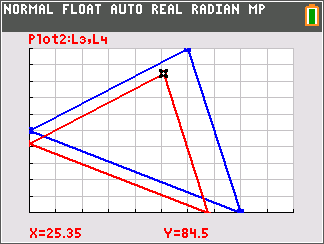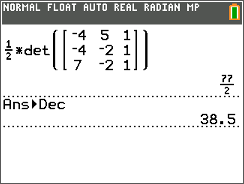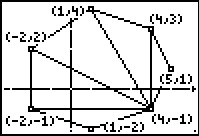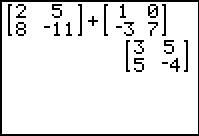### Dilations with Matrices

Students use matrices to perform dilations centered at the origin of triangles. Students will explore the effect of the scale factor on the size relationship between the preimage and image of a polygon.
•TI-84 Plus CE
•TI-84 Plus C Silver Edition
• TI-84 Plus Silver Edition
• TI-84 Plus### Triangle in the Matrix

Students represent a triangle with a matrix and use the determinant to find the area of three given triangles.
•TI-84 Plus CE
•TI-84 Plus C Silver Edition
• TI-84 Plus Silver Edition
• TI-84 Plus### Determining Area

Students use the area formula involving the determinant of a matrix.
•TI-84 Plus CE
•TI-84 Plus C Silver Edition
• TI-84 Plus Silver Edition
• TI-84 Plus••